;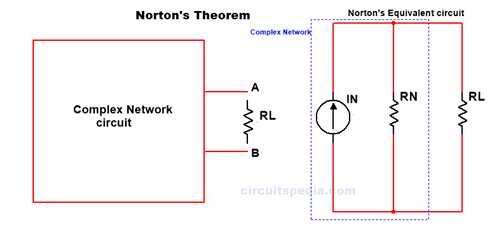## Norton's theorem statement

In Thevenin theorem, we see that in any complex circuit sources are converted in single voltage source Vth with the Series resistor Rth. Norton's theorem is also a method to convert any complex network into a simple circuit.

Norton Theorem, any two-terminal complicated network circuit can be converted in single Current source IN in parallel with a single resistor RN.

Actually in norton we convert any complex or complicted circuit into a simple circuit and I get current value across a Load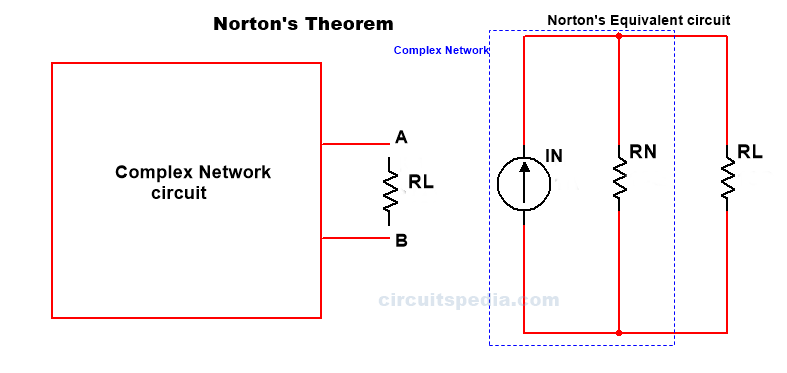### According to Norton theorem, Any linear bilateral network irrespective of its complexities can be reduced into Norton's equivalent circuit having a Norton short circuit current "Ir" in parallel with Norton's equivalent resistance Rn in parallel with load resistor RL.

What is Load resistor - Across which resistor asked to find the current that resistor is called Load resistor.

### Steps for solving the Norton theorem problems

Step1. Identify the load resistor RL.

Step 2.Replace RL by a short circuit at this place. The current flowing through this short circuit branch will be Norton's current IN. This current also is known as short-circuited current Isc.

Step 3. Remove the load resistance and replace all the active sources with their internal resistance.

Step 4. The equivalent resistance across the two open will be Norton's resistance RN.

Step 5. Draw Norton's equivalent circuit.

Step 6. Calculate IL using the identity IL=IN.RL/RN+RL

Example

Q.1 Find the value of current I in the given circuit using Norton's theorem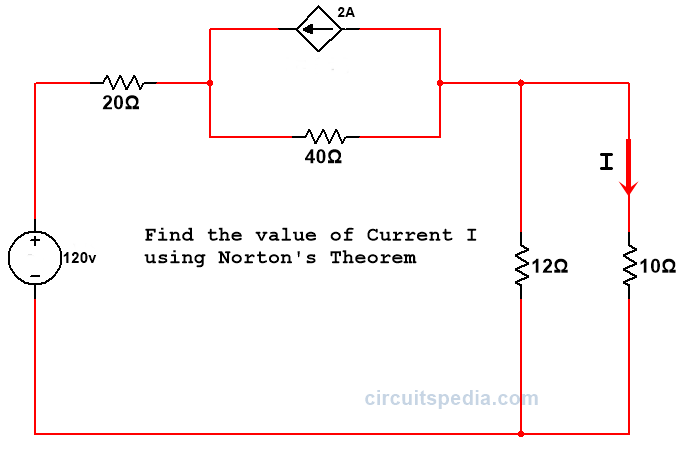Solution -

Here RL= 10Ω

Now I Replace the Load resistor with short circuit and Apply the KVL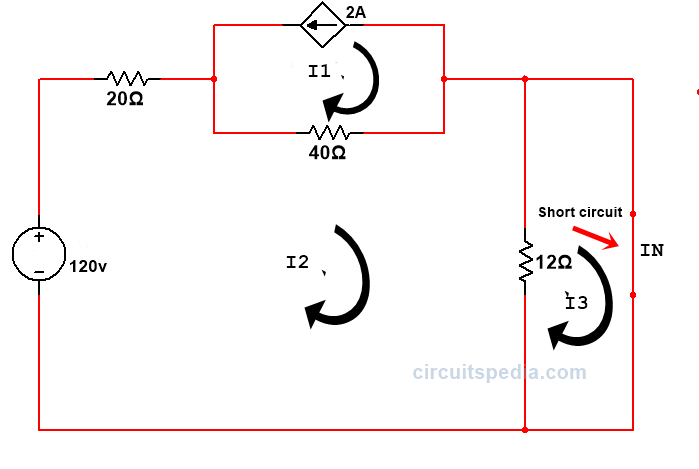I1=-2A

40I1-72I1+12I3=-120

Putting the value of I1

-80-72I2+12I3=-120

-72I1+12I3=-40   ----------Eq.1

And,  -12I3+12I2=0   ---------Eq.2

by solving the eq 1 & 2

I2=0.666A

Because of 10Ω resistor is short-circuited then 12Ω resistor also should be shorted, and then we don't need to find the current at IN.

Current I2 is passed across IN.

So,IN=I2=0.666A

Now we Remove the Load resistor RLK and repace all sources with their internal resistance.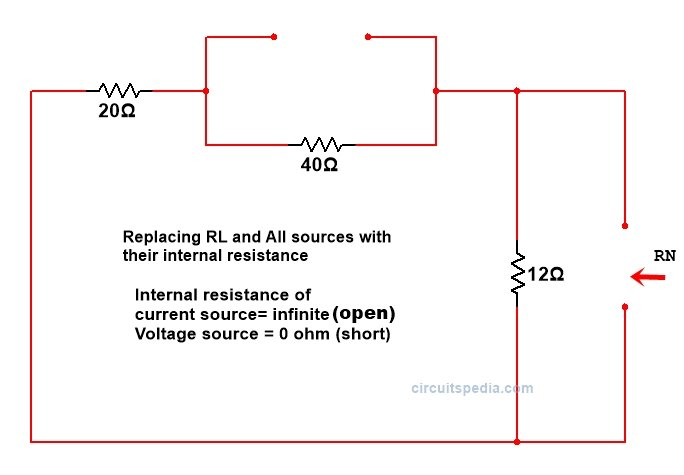RN=(20+40)//12

RN=60*12/60+12

RN=10Ω

by using Norton Identity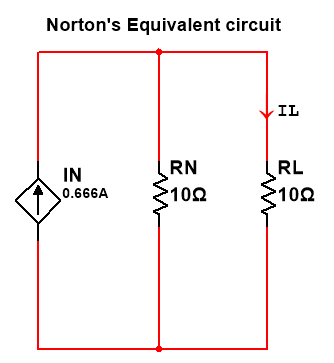IL=IN.RL/RN+RL

IL=0.333A (Ans)

## Q. 2 In the given network draw Norton's equivalent circuit and find the current through 18.75Ω resistor.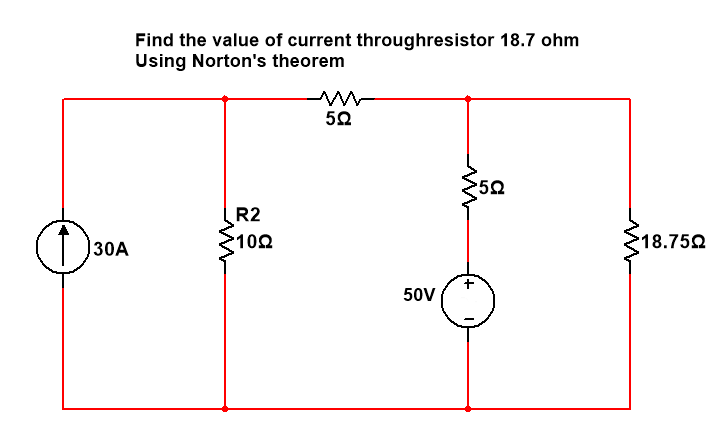Solution.-

Step 1-Short circuit the load resistance (RL)Step 2-Calculate the short circuit current IN or ISC.

I1=30A

I3= ISC or IN

(in mesh 2)  -10I1+20I2-5I3=-50  ------------eq.1

-10*30+20I2-5I3=-50

20I2-5I3=250

(In mesh 3)   -5I2+5I3=50  ----------eq. 2

by solving eq 1 & eq 2

I2=20A

I3=30A

I3=IN=30A

Step 3.-Remove all sources with internal resistance and Remove Load resistance RL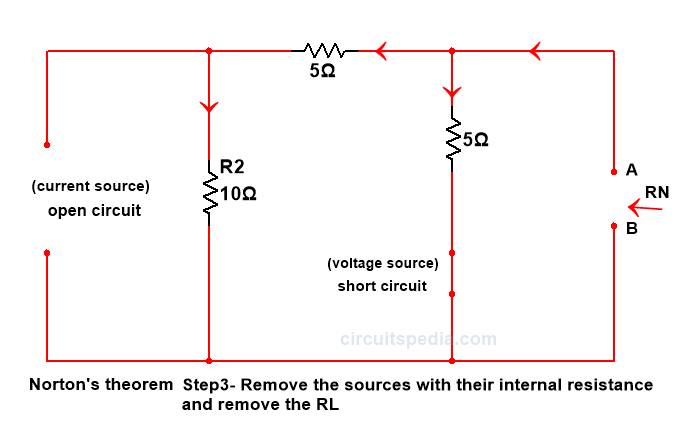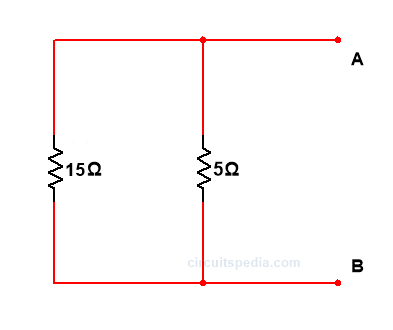RN=15*5/15+5 = 3.75

Step 4.-Draw norton equivalent circuit and calculate the IL using Identity IL=IN.RN/RN+RL30*3.75/3.75+18.75=5A

S0, IL=5A (Ans)